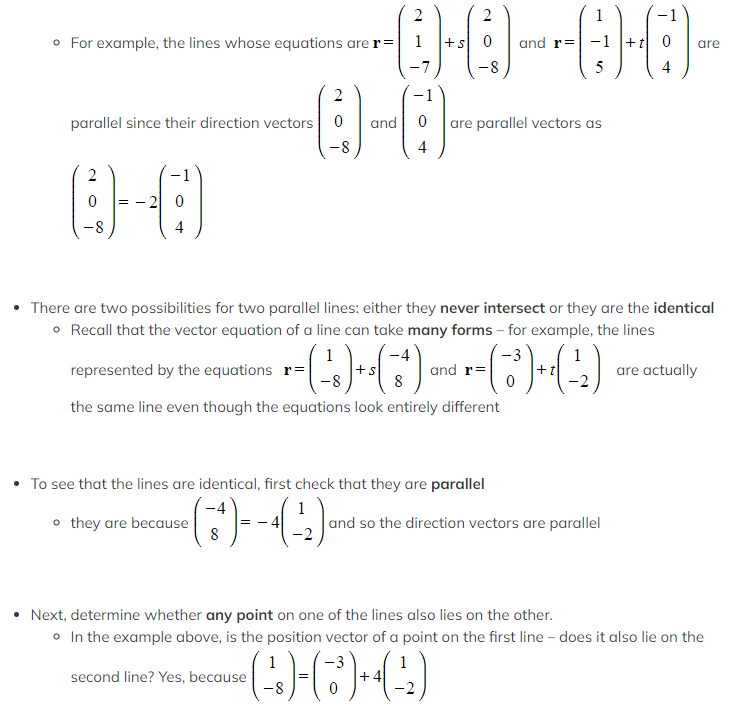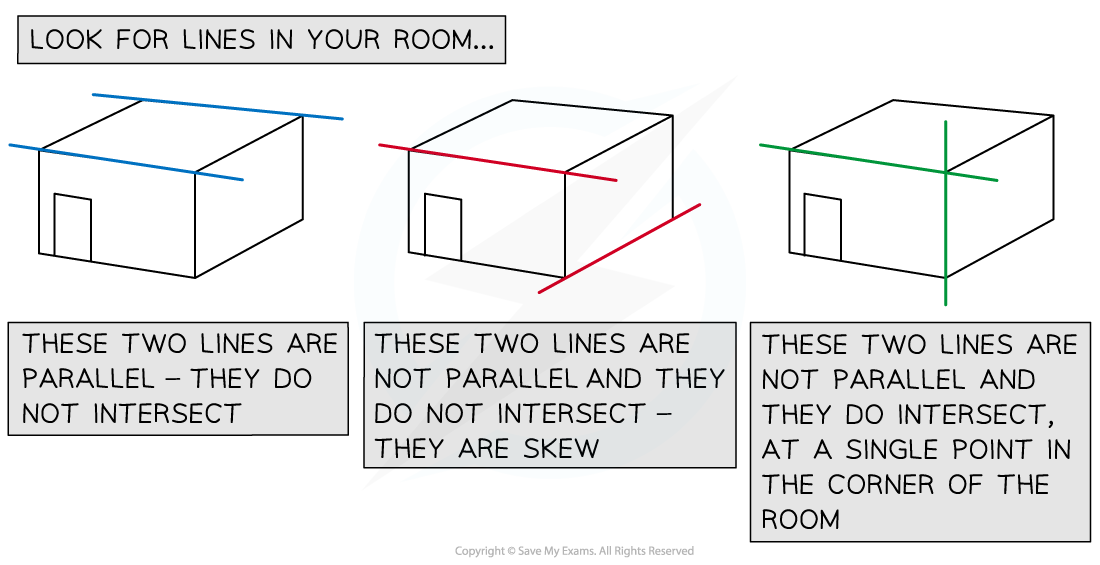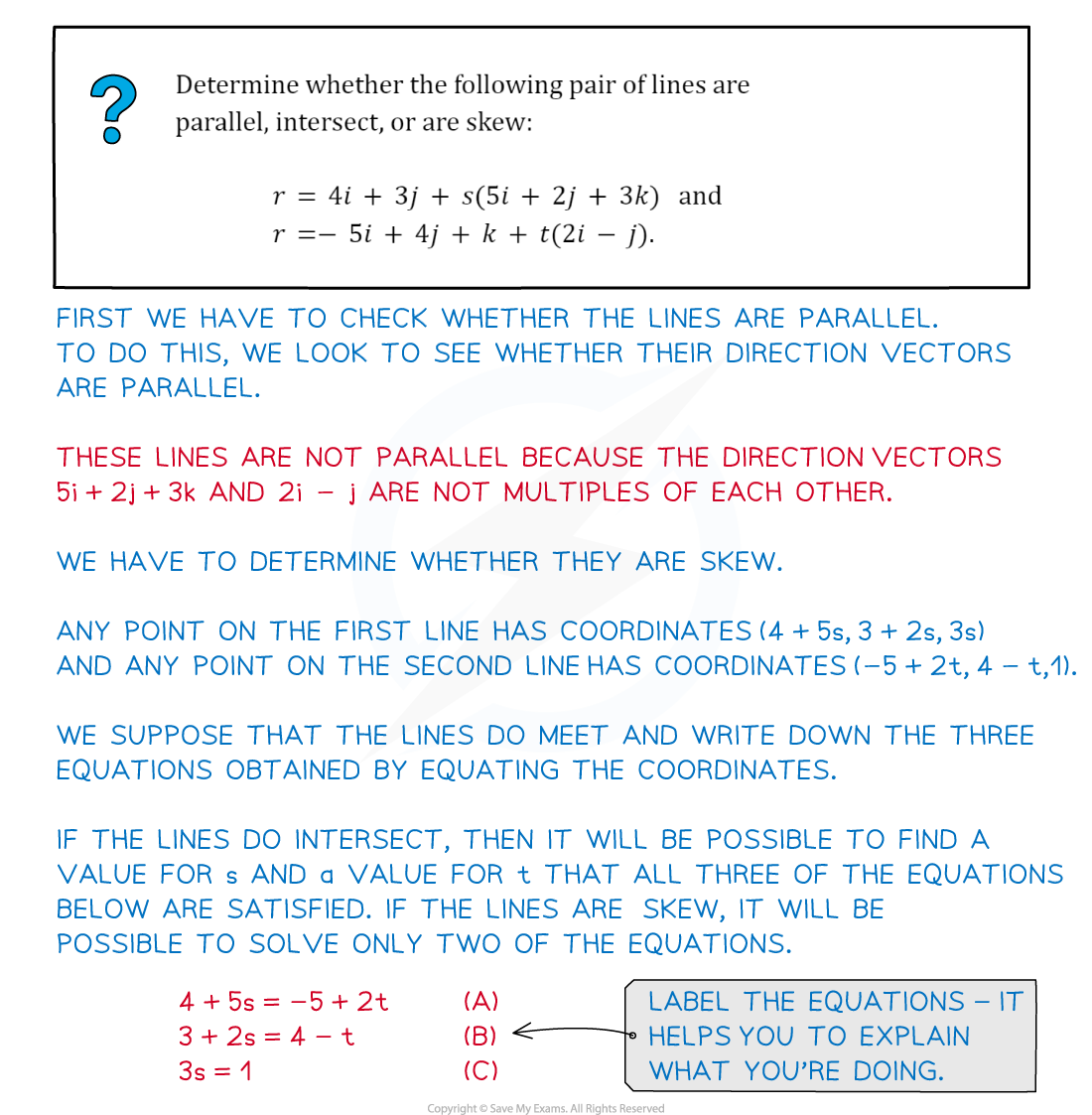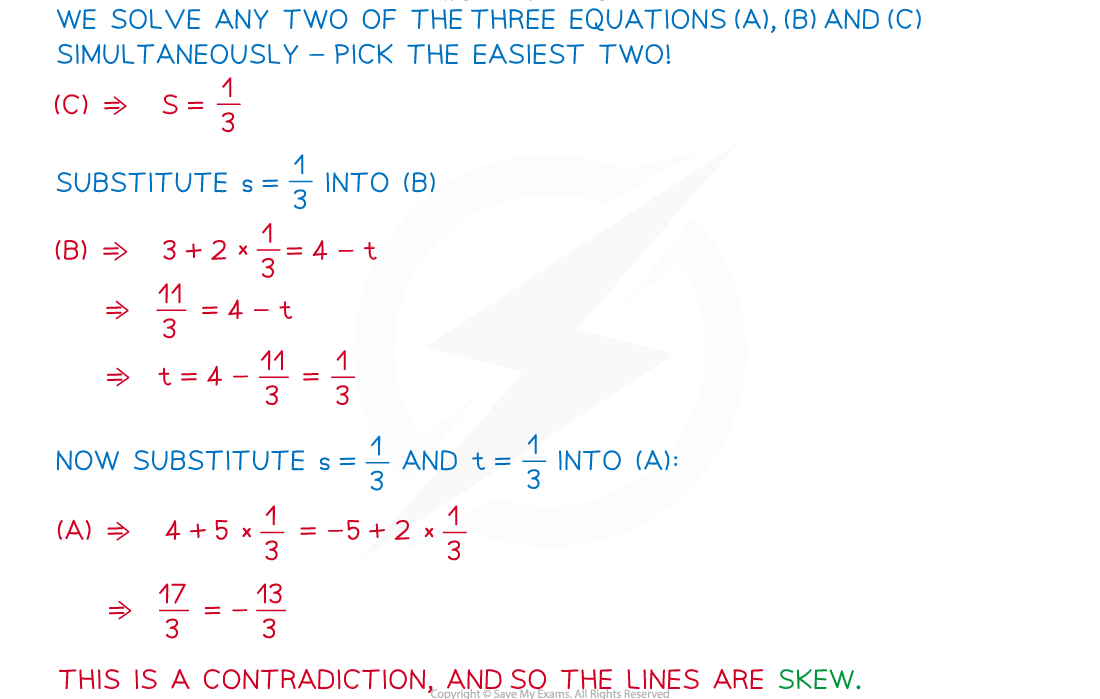# CIE A Level Maths: Pure 3复习笔记7.3.2 Parallel, Intersecting & Skew Lines

### Parallel, Intersecting & Skew Lines

In two dimensions, lines are either parallel or they intersect at a single point. If they are parallel, then either they have no points in common, or they share every point in common.

In three dimensions, there is a further possibility: a pair of lines might not be parallel and have no points of intersection. We say that the lines are skew.

#### How do I tell if two lines are parallel?

• Two lines are parallel if, and only if, their direction vectors are parallel
• This means the direction vectors will be scalar multiples of each other
•If two parallel lines share any point, then they share all points – i.e. they are identical

#### What are skew lines?

• First, start with another question: do lines which are not parallel necessarily intersect?
• In 2 dimensions, the answer is yes
• However, lines in 3 dimensions do not necessarily intersect
• Lines that are not parallel and which do not intersect are called skew lines#### How do I determine whether lines in 3D are parallel, skew, or intersecting?

• First, look to see if the direction vectors are parallel:
• if the direction vectors are parallel, then the lines are parallel
• if the direction vectors are not parallel, the lines are not parallel
• If the lines are parallel, check to see if they are identical:
• If they share any point, then they are identical
• If any point on one line is not on the other line, then the lines are not identical
• If the lines are not parallel, check whether they intersect:
• Using different letters, e.g. s and t, for the parameters, write down coordinates for a general point on each line
• Supposing that the lines do intersect: equate the two coordinates and write down three equations
• One for each component (i, j, k)
• Solve any two pairs of these equations simultaneously to find s and t
• Check whether the values of s and you have found satisfy the third equation
• If all three equations are satisfied, then the lines intersect
• If not all three equations are satisfied, then the lines are skew
• If a pair of lines are not parallel and do intersect, the unique point of intersection can be found by substituting the value of one of the parameters you have found into the coordinates for points on the appropriate line.

#### Worked Example#### Exam Tip

• Make sure that you use different letters, e.g. s and t, to represent the parameters in vector equations of different lines.